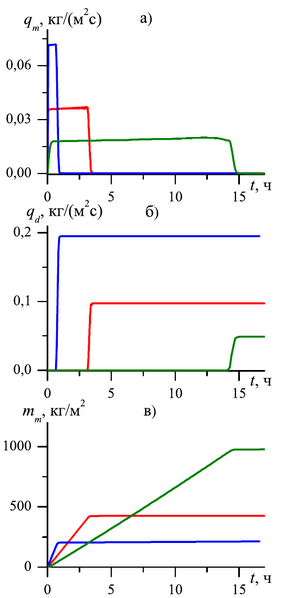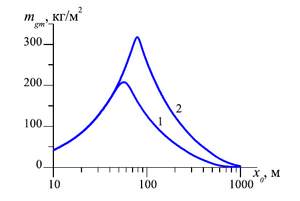ISSN 2658–5782
DOI 10.21662
Electronic Scientific Journalим. Р.Р. Мавлютова
УФИЦ РАНRafikova G.R., Khasanov M.K. Analysis of methane production intensity during its displacement from a gas hydrate formation by carbon dioxide. Multiphase Systems. 14 (2019) 3. 149–156 (in Russian).
2019. Vol. 14. Issue 3, Pp. 149–156
URL: http://mfs.uimech.org/mfs2019.3.021,en
DOI: 10.21662/mfs2019.3.021
Analysis of methane production intensity during its displacement from a gas hydrate formation by carbon dioxide
Rafikova G.R.∗,∗∗, Khasanov M.K.
Sterlitamak Branch of Bashkir State University, Sterlitamak
∗∗Mavlyutov Institute of Mechanics, Ufa

### Abstract

The theoretical model is considered in the one-dimensional approximations and numerical solutions are obtained for the process of replacing methane with carbon dioxide from a hydrate in a formation saturated with methane and its hydrate when carbon dioxide is injected into the formation. The process is considered under thermobaric conditions corresponding to the stability region of methane gas and carbon dioxide and the region of existence of CO2 in the form of a gaseous phase. The case is considered when the rate of carbon dioxide hydrate formation is limited by diffusion of carbon dioxide through the formed hydrate layer between the gas mixture stream and methane hydrate. It is accepted that the hydration substitution process occurs without the release of water from the hydrate. To describe the mathematical model, the main equations are the mass conservation equations for methane, carbon dioxide and their hydrates, Darcy’s law for filtration, Fick’s law for diffusive mixing of the gas mixture, state equations for the gas phase, Dalton’s law, energy equation, diffusion equation for transport CO2 through the hydration layer at the pore microchannel scale. The dynamics of the mass flow rates of the outgoing carbon dioxide and methane recovered has been investigated. The influence of the diffusion coefficient, the absolute permeability and the length of the formation on the intensity of the methane produced as a result of the gas substitution process is analyzed. Three main stages of the process were identified: displacement of free methane from the reservoir; extraction of free methane obtained as a result of the beginning of hydrate substitution in the formation; complete conversion of methane hydrate to carbon dioxide hydrate and complete extraction of methane from the formation. It is determined how the two main factors relate to each other in terms of the degree of influence on the replacement rate: heat and mass transfer in the reservoir and the kinetics of the replacement process.

Keywords

replacement of methane with carbon dioxide from the hydrate,
gas hydrate formation,
mass flow rate,
hydration substitution kinetics,
filtration mass transfer

## Article outline

Purpose: The theoretical study of the process of replacing methane with carbon dioxide from the gas hydrate in a formation saturated with methane and its hydrate, when the formation is purged with carbon dioxide.

One of the most well-known and promising methods for the extraction of methane from a gas hydrate formation is the depressive and thermal effects on the formation, the introduction of inhibitors into the formation. The last of the methods considered and poorly studied is the injection of carbon dioxide into the layers saturated with methane and its hydrate. The essence of the method is that carbon dioxide gas hydrate is more stable than methane gas hydrate and carbon dioxide molecules displace methane molecules from the gas hydrate. The advantages of this method are the utilization of greenhouse gas and the preservation of the mechanical strength of the rock. Also, if the decomposition of gas hydrate during depression and heating takes place with the absorption of heat, then in this case the process will occur with a small amount of heat.

Methods: For describing the mathematical model of the displacement of methane from a gas hydrate formation by carbon dioxide, methods and equations of the mechanics of multiphase media were applied. For constructing the mathematical model, we used the basic equations of mass conservation for methane, carbon dioxide and their hydrates, the Darcy law for describing the flow in the reservoir, the Fick law for diffusive mixing of the gas mixture, the equation of state for the gas phase, the Dalton law for determining the pressure of the system, the heat equation for determining changes in the energy released as a result of phase transitions and filtration in the reservoir, the diffusion equation for gas transfer through the gashydrate layer. We have obtained a quasistationary analytical solution for the diffusion equation. The density of mobile carbon dioxide in the hydrate was determined. The numerical implementation of the solution of the basic system of equations is carried out using an explicit finite-difference scheme.

As a result of the study, it was determined that in order to change the mass flow rate of methane produced through the external boundary of the formation over time, three stages can be distinguished:

1. At the first stage free methane, which is in the initial state in the pores of the formation, is displaced. This stage is accompanied by a sharp increase in methane consumption at the outlet of the reservoir.
2. The second stage is characterized by the displacement of methane obtained as a result of the process of substitution of methane from the composition of the hydrate with carbon dioxide. At this stage, the value of the mass flow rate of methane produced through the external boundary of the formation is approximately stable over time.
3. The third stage is characterized by the complete transition of methane hydrate to carbon dioxide hydrate and the complete extraction of methane from the gas hydrate formation, which corresponds to a decrease in the mass flow of methane and an increase in the mass flow of carbon dioxide.

Conclusions: The duration of the second stage of the process increases with a decrease of permeability of the reservoir. The period of the second stage of the process decreases and the third stage increases with a decrease of diffusion coefficient of carbon dioxide transfer through the gas hydrate layer. This events is associated with a decrease of the effect of gas hydrate substitution kinetics and an increase of the effect of filtration transfer in the formation.

## References

1. Vasil’ev V.I., Popov V.V., Tsypkin G.G. Numerical investigation of the decomposition of gas hydrates coexisting with gas in natural reservoirs // Fluid Dynamics. 2006. V. 41. No. 4. Pp. 599–605.
DOI: 10.1007/s10697-006-0078-z
2. Tsypkin G.G. Techeniya s fazovymi perekhodami v poristykh sredakh. M.: Fizmatlit. 2009. P. 232 (in Russian).
3. Nazmutdinov F.F., Khabibullin I.L. Mathematical modeling of gas desorption from a gas hydrate // Fluid Dynamics. 1996. V.31. No. 5. Pp. 724–730.
https://www.elibrary.ru/item.asp?id=28097978
4. Shagapov V.Sh., Musakayev N.G. Dinamika obrazovaniya i razlozheniya gidratov v sistemakh dobychi, transportirovki i khraneniya gaza. M.: Nauka. 2016. P. 238 (in Russian).
5. Moridis G.J. Numerical studies of gas production from methane hydrates // Society of Petroleum Engineers Journal. Vol. 8, No 4.
DOI: 10.2118/87330-PA
6. Espinoza N.D., Santamarina C.J. P-wave monitoring of hydratebearing sand during CH4–CO2 replacement // Int. J. Greenhouse Gas Control. 2011. Vol. 5, P. 1031–1038.
DOI: 10.1016/j.ijggc.2011.02.006
7. Jung J.W., Santamarina J.C. CH4-CO2 replacement in hydratebearing sediments: A pore-scale study // Geochemistry, Geophysics, Geosystems. 2010. Vol. 11. Article Q0AA13.
DOI: 10.1029/2010GC003339
8. Jung J.W., Espinoza D.N., Santamarina J.C. Properties and phenomena relevant to CH42 replacement in hydratebearing sediments // J. Geophysical Research: Solid Earth. 2010. Vol. 115. Article B10102.
DOI: 10.1029/2009JB000812
9. Falenty A., Qin J., Salamatin A.N., Yang L., Kuhs W.F. Fluid composition and kinetics of the in situ replacement in CH–CO2 hydrate system // Journal of physical chemistry C. 2016. Vol. 120, No 48. P. 27159–27172.
DOI: 10.1021/acs.jpcc.6b09460
10. Tsypkin G.G. Formation of hydrate in injection of liquid carbon dioxide into a reservoir saturated with methane and water // Fluid Dynamics. 2016. V. 51, No. 5. Pp. 672–679.
DOI: 10.1134/S0015462816050112
11. Khasanov M.K. Injection of boiling liquid carbon dioxide into a stratum, accompanied by replacement of methane in hydrate by carbon dioxide // Journal of Applied Mathematics and Mechanics. 2016. V. 80, No. 5. Pp. 391–399.
DOI: 10.1016/j.jappmathmech.2017.02.006
12. Musakayev N.G., Khasanov M.K. [The mathematical model of the carbon dioxide burial in the reservoir saturated with hydrate] Trudy Instituta mekhaniki im. R.R. Mavlyutova Ufimskogo nauchnogo tsentra RAN [Proceedings of the Institute of Mechanics. R.R. Mavlyutov Ufa Scientific Center, Russian Academy of Sciences]. 2016. V. 11, No. 2. Pp. 181-–187 (In Russian).
DOI: 10.21662/uim2016.2.026
13. Shepelkevich O.F [The replacement of methane hydrate in the reservoir by injection into the liquid carbon dioxide] Trudy Instituta mekhaniki im. R.R. Mavlyutova Ufimskogo nauchnogo tsentra RAN [Proceedings of the Institute of Mechanics. R.R. Mavlyutov Ufa Scientific Center, Russian Academy of Sciences]. 2017. V. 12, No. 2. Pp.206–213 (In Russian).
DOI: 10.21662/uim2017.2.031
14. Shagapov V.Sh., Khasanov M.K., Bayramgulova R.S. [The theory of injection of liquid carbon dioxide in formation, saturated system «hydrate of methane–methane» in the mode of formation of the intermediate melt zone] Trudy Instituta mekhaniki im. R.R. Mavlyutova Ufimskogo nauchnogo tsentra RAN [Proceedings of the Institute of Mechanics. R.R. Mavlyutov Ufa Scientific Center, Russian Academy of Sciences]. 2016. V. 11, No. 2. Pp. 171–180 (In Russian).
DOI: 10.21662/uim2016.2.025
15. Shagapov V.Sh., Rafikova G.R., Khasanov M.K. The theory of the replacement of methane by carbon dioxide in gas hydrates // Theoretical foundations of chemical engineering. 2019. V. 53, No. 1. Pp. 64–74.
DOI: 10.1134/S0040579518060118
16. Shagapov V.Sh., Khasanov M.K., Rafikova G.R. [Displacement of methane from a gas hydrate reservoir in the process of carbon dioxide injection] [Vestnik tomskogo gosudarstvennogo universiteta-matematika i mekhanika-tomsk state university journal of mathematics and mechanics]. 2016. No. 6. Pp. 104–114 (In Russian).
DOI: 10.17223/19988621/44/9
17. Musakaev N.G., Khasanov M.K., Rafikova G.R. Mathematical model of the methane replacement by carbon dioxide in the gas hydrate reservoir taking into account the diffusion kinetics // AIP Conference Proceedings. 2018. Is. 1939. P. 020034-1–020034-6.
DOI: 10.1063/1.5027346
18. Makogom Yu.F. [Gazogidraty. Istoriya izucheniya i perspektivy osvoyeniya] [Geologiya i poleznyye iskopayemyye Mirovogo okeana]. 2010. No. 2. Pp. 5–21 (In Russian).
https://elibrary.ru/item.asp?id=15122639
19. Shagapov V.S., Chiglintseva A.S., Rafikova G.R. On the applicability of a quasi-stationary solution of the diffusion equation for the hydrate layer formed at the gas–ice (water) interface // Theoretical Foundations of Chemical Engineering. 2018. V. 52. No. 4. Pp. 560–567.
DOI: 10.1134/S0040579518040413
20. Shagapov V.Sh., Rafikova G.R., Khasanov M.K. On the theory of formation of gas hydrate in partially water-saturated porous medium when injecting methane // High Temperature. 2016. V. 54, No. 6. Pp. 858—866.
DOI: 10.1134/S0018151X16060171